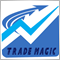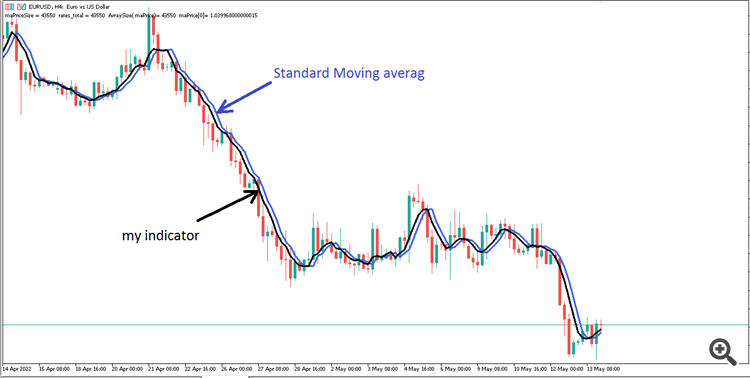# Moving Average by iMAOnArray in Mql51103

Hello

I tried to write the equivalent for the iMAOnArray function in MetaTrader 4 using MovingAverages.mqh in MetaTrader 5. The problem is obvious in the attached photos. The result differs from the Moving averag indicator. It is also not calculated for period 1.code:

```//+------------------------------------------------------------------+
//|                                           Moving_Avrag_close.mq5 |
//|                                             https://www.mql5.com |
//+------------------------------------------------------------------+
#property version   "1.00"
#property indicator_chart_window
#property description "Moving Avrag close"

#include <MovingAverages.mqh>

//--- indicator settings
#property indicator_chart_window
#property indicator_buffers 2
#property indicator_plots   1
#property indicator_type1   DRAW_LINE
#property indicator_color1  clrRed
#property indicator_label1  "Moving Avrag"

//---- enum

//---- parameters
input int             MaPeriod  = 10;           // Period
input ENUM_MA_METHOD  MaMetod   = MODE_SMA;     // Metod
//--------------------------------------------------------------

//--- indicator buffers
double LineBuffer[],maPrice[];

//+------------------------------------------------------------------+
//| Custom indicator initialization function                         |
//+------------------------------------------------------------------+
int OnInit()
{

//--- indicator buffers mapping
SetIndexBuffer(0,LineBuffer,INDICATOR_DATA);
SetIndexBuffer(1,maPrice,INDICATOR_CALCULATIONS);
//---
IndicatorSetInteger(INDICATOR_DIGITS,_Digits);
//--- sets first bar from what index will be drawn
IndicatorSetString(INDICATOR_SHORTNAME,"Moving Avrag close");
//--- sets drawing line empty value
PlotIndexSetDouble(0,PLOT_EMPTY_VALUE,0.0);
//---
//--- first calculation or number of bars was changed
ArrayInitialize(LineBuffer,0.0);
ArrayInitialize(maPrice ,0.0);
return(INIT_SUCCEEDED);
}
//+------------------------------------------------------------------+
//| Custom indicator iteration function                              |
//+------------------------------------------------------------------+
int OnCalculate(const int rates_total,
const int prev_calculated,
const datetime &time[],
const double &open[],
const double &high[],
const double &low[],
const double &close[],
const long &tick_volume[],
const long &volume[],
{
//---
//--- check for data
if(rates_total<MaPeriod )
return(0);

if(prev_calculated<0)
return(0);

//--- check for bars count
int Counted = MathMax(prev_calculated-1,0);
Counted = MathMax(Counted ,MaPeriod );

//--- first calculation of bars
if(prev_calculated==0)
{
for (int i=0 ; i<Counted && !_StopFlag; i++)
{
LineBuffer[i] = close[i];
maPrice[i]= close[i];
}
}

//----First Cycle----
int maPriceSize = iMAOnArray(close,rates_total,prev_calculated,MaPeriod,MaMetod,0,maPrice);
Comment("maPriceSize = " ,maPriceSize ,"  rates_total = ",rates_total,"  ArraySize( maPrice)= ",ArraySize( maPrice),"  maPrice= ",maPrice[rates_total-1]);

//----main Cycle-----
for(int i=Counted ; i<rates_total && !_StopFlag; i++)
{
LineBuffer[i] = maPrice[i];
}

//--- return value of prev_calculated for next call
return(rates_total);
}
//+------------------------------------------------------------------+

//+------------------------------------------------------------------+
//|  iMA On Array                                                    |
//+------------------------------------------------------------------+
int iMAOnArray(double const &array[],
int rates_total,
int prev_calculated,
int period,
int ma_method,
int begin,
double &Extern_array[]
)
{
switch(ma_method)
{
case MODE_EMA:
{int X=ExponentialMAOnBuffer(rates_total,prev_calculated,begin,period,array,Extern_array);return(X);}
break;
case MODE_LWMA:
{int X=LinearWeightedMAOnBuffer(rates_total,prev_calculated,begin,period,array,Extern_array);return(X);}
break;
case MODE_SMA:
{int X=SimpleMAOnBuffer(rates_total,prev_calculated,begin,period,array,Extern_array);return(X);}
break;
case MODE_SMMA:
{int X=SmoothedMAOnBuffer(rates_total,prev_calculated,begin,period,array,Extern_array);return(X);}
break;

default: return(0);
break;
}
return(0);
}
```27545

`           LineBuffer[i] = close[i];`

The buffer is as-series. You didn't set your array likewise.

To define the indexing direction in the time[], open[], high[], low[], close[], tick_volume[], volume[] and spread[] arrays, call the ArrayGetAsSeries() function. In order not to depend on defaults, call the ArraySetAsSeries() function for the arrays to work with.
OnCalculate - Event Handling - MQL5 Reference - Reference on algorithmic/automated trading language for MetaTrader 51103

William Roeder #:

The buffer is as-series. You didn't set your array likewise.

I changed the code as follows but the result did not change.

```//+------------------------------------------------------------------+
//|                                           Moving_Avrag_close.mq5 |
//|                                             https://www.mql5.com |
//+------------------------------------------------------------------+
#property version   "1.00"
#property indicator_chart_window
#property description "Moving Avrag close"

#include <MovingAverages.mqh>

//--- indicator settings
#property indicator_chart_window
#property indicator_buffers 2
#property indicator_plots   1
#property indicator_type1   DRAW_LINE
#property indicator_color1  clrRed
#property indicator_label1  "Moving Avrag"

//---- enum

//---- parameters
input int             MaPeriod  = 10;           // Period
input ENUM_MA_METHOD  MaMetod   = MODE_SMA;     // Metod
//--------------------------------------------------------------

//--- indicator buffers
double LineBuffer[],maPrice[];

//+------------------------------------------------------------------+
//| Custom indicator initialization function                         |
//+------------------------------------------------------------------+
int OnInit()
{

//--- indicator buffers mapping
SetIndexBuffer(0,LineBuffer,INDICATOR_DATA);
SetIndexBuffer(1,maPrice,INDICATOR_CALCULATIONS);
//---
IndicatorSetInteger(INDICATOR_DIGITS,_Digits);
//--- sets first bar from what index will be drawn
IndicatorSetString(INDICATOR_SHORTNAME,"Moving Avrag close");
//--- sets drawing line empty value
PlotIndexSetDouble(0,PLOT_EMPTY_VALUE,0.0);
//---
//--- first calculation or number of bars was changed
ArrayInitialize(LineBuffer,0.0);
ArrayInitialize(maPrice ,0.0);
return(INIT_SUCCEEDED);
}
//+------------------------------------------------------------------+
//| Custom indicator iteration function                              |
//+------------------------------------------------------------------+
int OnCalculate(const int rates_total,
const int prev_calculated,
const datetime &time[],
const double &open[],
const double &high[],
const double &low[],
const double &close[],
const long &tick_volume[],
const long &volume[],
{
//---
ArraySetAsSeries( LineBuffer, true);
ArraySetAsSeries( maPrice, true);
ArraySetAsSeries( time, true);
ArraySetAsSeries( open, true);
ArraySetAsSeries( high, true);
ArraySetAsSeries( low, true);
ArraySetAsSeries( close, true);
ArraySetAsSeries( tick_volume, true);
ArraySetAsSeries( volume, true);

Comment(
"LineBuffer: ",ArrayGetAsSeries(LineBuffer),
"  maPrice: ",ArrayGetAsSeries(maPrice),

"  time: ",ArrayGetAsSeries(time),
"  open: ",ArrayGetAsSeries(open),
"  high: ",ArrayGetAsSeries(high),
"  low: ",ArrayGetAsSeries(low),
"  close: ",ArrayGetAsSeries(close),

"  tick_volume: ",ArrayGetAsSeries(tick_volume),
"  volume: ",ArrayGetAsSeries(volume),
);

//--- check for data
if(rates_total<MaPeriod )
return(0);

if(prev_calculated<0)
return(0);

//--- check for bars count
int limit = (rates_total-1) - prev_calculated;
if (limit<0) limit = 0;

int Start = rates_total-MaPeriod;
limit=MathMin(limit,Start);

//--- first calculation of bars
if(prev_calculated==0)
{
for (int i=rates_total-1 ; i>limit && !_StopFlag; i--)
{
LineBuffer[i] = close[i];
maPrice[i]= close[i];
}
}

int maPriceSize = iMAOnArray(close,rates_total,prev_calculated,MaPeriod,MaMetod,0,maPrice);

//----main Cycle-----
for(int i=limit ; i>=0 && !_StopFlag; i--)
{
LineBuffer[i] = maPrice[i];
}

//--- return value of prev_calculated for next call
return(rates_total);
}
//+------------------------------------------------------------------+

//+------------------------------------------------------------------+
//|  iMA On Array                                                    |
//+------------------------------------------------------------------+
int iMAOnArray(double const &array[],
int rates_total,
int prev_calculated,
int period,
int ma_method,
int begin,
double &Extern_array[]
)
{
switch(ma_method)
{
case MODE_EMA:
{int X=ExponentialMAOnBuffer(rates_total,prev_calculated,begin,period,array,Extern_array);return(X);}
break;
case MODE_LWMA:
{int X=LinearWeightedMAOnBuffer(rates_total,prev_calculated,begin,period,array,Extern_array);return(X);}
break;
case MODE_SMA:
{int X=SimpleMAOnBuffer(rates_total,prev_calculated,begin,period,array,Extern_array);return(X);}
break;
case MODE_SMMA:
{int X=SmoothedMAOnBuffer(rates_total,prev_calculated,begin,period,array,Extern_array);return(X);}
break;

default: return(0);
break;
}
return(0);
}
```1103

Is there anyon to guide?